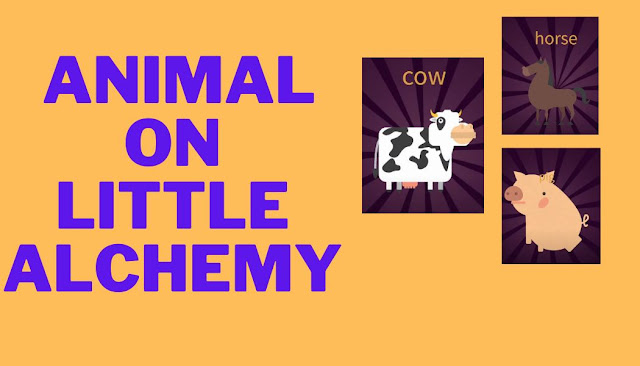How to make wild animal on little alchemy

## How to make wild animal on little alchemy

### 1. How to make a cow on little alchemy

• FIRE + FIRE = ENERGY
• EARTH + FIRE = LAVA
• LAVA + AIR = STONE
• FIRE + STONE = METAL
• METAL + STONE = PLOW
• EARTH + PLOW = FIELD
• WATER + WATER = PUDDLE
• PUDDLE  + WATER = POND
• POND + WATER = LAKE
• LAKE + WATER = SEA
• SEA + EARTH = PRIMORDIAL SOUP
• PRIMORDIAL SOUP + ENERGY =  LIFE
• EARTH + EARTH = LAND
• LAND + LIFE = ANIMAL AND SOIL
• ANIMAL  + FIELD = LIVESTOCK AND HORSE
• LIVESTOCK + FIELD  = COW

### 2. How to make a horse little alchemy

• FIRE + FIRE = ENERGY
• EARTH + FIRE = LAVA
• LAVA + AIR = STONE
• FIRE + STONE = METAL
• METAL + STONE = PLOW
• EARTH + PLOW = FIELD
• WATER + WATER = PUDDLE
• PUDDLE  + WATER = POND
• POND + WATER = LAKE
• LAKE + WATER = SEA
• SEA + EARTH = PRIMORDIAL SOUP
• PRIMORDIAL SOUP + ENERGY =  LIFE
• EARTH + EARTH = LAND
• LAND + LIFE = ANIMAL AND SOIL
• ANIMAL  + FIELD = LIVESTOCK AND HORSE

### 3. How to make a pig little alchemy

• EARTH = WATER = MUD
• EARTH + EARTH = LAND
• FIRE + FIRE = ENERGY
• WATER + WATER = PUDDLE
• PUDDLE + WATER = POND
• POND + WATER = LAKE
• LAKE + WATER = SEA
• SEA + EARTH = PREMORDIAL SOUP
• PREMORDIAL SOUP + ENERGY = LIFE
• LIFE + LAND = ANIMANL AND SOIL
• ANIMANL  + MUD = PIG